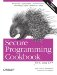# Converting Between Numeric Types

Problem

You have number of one type and you need to convert it to another, such as an int to a short or a vice versa, but you want to catch any overflow or underflow errors at runtime.

Solution

Use Boost's numeric_cast class template. It performs runtime checks that throw an exception of type bad_numeric_cast if you will overflow or underflow the variable where you are putting a value. Example 3-8 shows you how to do this.

Example 3-8. Safe numeric conversions

```#include
#include

using namespace std;
using boost::numeric_cast;

int main( ) {

// Integer sizes
try {
int i = 32767;
short s = numeric_cast(i);

cout << "s = " << s << endl;

i++; // Now i is out of range (if sizeof(short) is 2)
s = numeric_cast(i);
}
cerr << e.what( ) << endl;
}

try {
int i = 300;
unsigned int ui = numeric_cast(i);

cout << ui << endl; // Fine

i *= -1;
ui = numeric_cast(i); // i is negative!
}
cerr << e.what( ) << endl;
}

try {
double d = 3.14;
int i = numeric_cast(d);

i = numeric_cast(d); // This shaves off the 0.14!

cout << i << endl; // i = 3

}
cerr << e.what( ) << endl;
}
}```

Discussion

You are probably aware of the fact that the basic C++ types have different sizes. The C++ standard has strict specifications for the relative size of typesan int is always at least as big as a short int--but it does not specify the absolute size. What this means is that if you take a long int and try to put it in a short, or attempt to put an int in an unsigned int, then you may be losing information about the value in the source variable, such as its sign or even part of its numeric value.

Just knowing that this causes problems isn't enough. You may have tight space requirements and not want to use four bytes for a long when you can get away with two for a short (if your platform, in fact, uses these sizes, which are common but not guaranteed). Because of your space requirements, you want to try to store values in the smallest possible type. If you want to live dangerously but want a safety net, use Boost's numeric_cast to catch loss of data at runtime.

The syntax of numeric_cast is straightforward. It is a function template, declared like this:

```template
inline Target numeric_cast(Source arg)```

It is just like lexical_cast if you have already read Recipe 3.1 or Recipe 3.3. There are two template parameters, Target and Source, which represent the types of the originating and destination values. Because it is a function template, the compiler can deduce the type of the Source template argument, so you only need to supply Target, like this:

```int i = 32767;
short s = numeric_cast(i);```

short is the template argument for the Target parameter. The compiler figures out that Source is an int because i is an int.

In this case, I am cramming an int into a short. On my (Windows XP) system, an int is four bytes and a short is two. A short is signed, which means that I have 15 bits to represent a number with and, therefore, 32,767 is the maximum positive value it can hold. The above piece of code goes off without a hitch, but when I increment i by one, it goes beyond the range of a short:

```i++;
s = numeric_cast(i); // Uh-oh```

And a bad_numeric_cast exception is thrownyou get the idea. See the rest of Example 3-8: numeric_cast also catches underflow if you try to assign a negative signed value to an unsigned type.

But numeric_cast doesn't solve all of your problems. If you try to put a floating-point value in a nonfloating-point type, you lose everything to the right of the decimal, correct? numeric_cast does not help you with this, so don't think that it can rescue you from all of your risky endeavors. For example, consider this piece of code from Example 3-8:

```double d = 3.14;
int i = numeric_cast(d); // Ouch```

No exception is thrown here. But it is if you try this:

```double d = -3.14;
unsigned int ui = numeric_cast(d);```

Because regardless of you tossing everything to the right of the decimal point out the window, you are losing the negative sign, and that is bad.

Recipe 3.1 and Recipe 3.3Secure Programming Cookbook for C and C++: Recipes for Cryptography, Authentication, Input Validation & More
ISBN: 0596003943
EAN: 2147483647
Year: 2006
Pages: 241